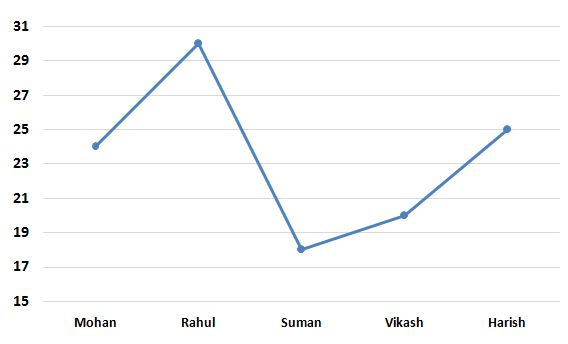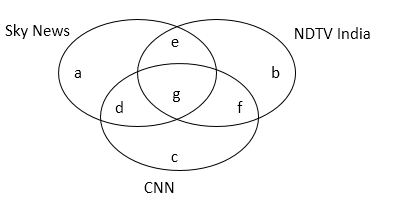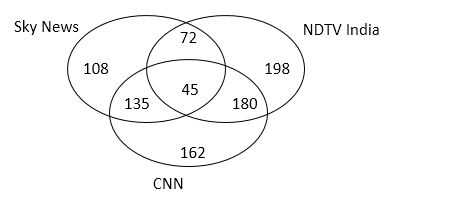# SBI PO Quantitative Aptitude Questions 2019 (Day-30) High Level New Pattern

SBI PO 2019 Notification is about to come and it is the most awaited exam among the aspirants. We all know that new pattern questions are introducing every year in the SBI PO exam. Further, the questions are getting tougher and beyond the level of the candidate’s expectations.

Our IBPS Guide is providing High-Level New Pattern Quantitative Aptitude Questions for SBI PO 2019 so the aspirants can practice it on a daily basis. These questions are framed by our skilled experts after understanding your needs thoroughly. Aspirants can practice these high-level questions daily to familiarize with the exact exam pattern. We wish that your rigorous preparation leads you to a successful target of becoming SBI PO.

[WpProQuiz 5440]

### Click Here for SBI PO Pre 2019 High-Quality Mocks Exactly on SBI Standard

Directions (1 – 5): Study the following information carefully and answer the questions given below:

The following line graph represents present ages (in years) of five boys:The following table represents ratio of the present ages of five boys and five girls.1) Average of the ages of Rahul, Sarita, Nimo and Ragini after two years will be 28.5 years. Ragini is two years older than Nimo. Average age of Nimo and Nidhi before four years was 21 years. Present age of Nidhi is what percent of the present age of Harish?

a) 96%

b) 88%

c) 92%

d) 76%

e) None of these

2) Respective ratio of the ages of Mohan and Nitin after six years will be 15: 17 and respective ratio of the ages of Vinita and Sumi before five years was 4: 3. Sum of the present ages of Mohan and Sumi is approximately what percent of the sum of the present ages of Nitin and Vinita?

a) 71%

b) 83%

c) 79%

d) 69%

e) 87%

3) Respective ratio of the ages of Parul and Nisha after four years will be 12: 11. Average of the present ages of Nisha, Tina and Vinay is 21 years. If Vinay is three years older than Shashank, find the average of the present ages of Parul and Shashank.

a) 19 years

b) 17 years

c) 21 years

d) 23 years

e) None of these

4) Find the relation between following two quantities:

Quantity I: Average of the present ages of Suman, Neha and Merlin is 24 years. Find the difference between the present ages of Rahul and Merlin.

Quantity II: Average of the present ages of Vikash, Sarita and Kavita is 22 years. Find the difference between the present ages of Mohan and Kavita.

a) Quantity I > Quantity II

b) Quantity I < Quantity II

c) Quantity I ≥ Quantity II

d) Quantity I ≤ Quantity II

e) Quantity I = Quantity II or no relation can be established.

5) Find the age of Seema after four years.

Statement I: Average of the present ages of Vinita, Tina and Deepa is 24 years. Deepa is two years younger than Meeta.

Statement II: Average of the ages of Meeta and Seema before two years was 22 years.

a) Statement I alone is sufficient to answer the question, but the statement II alone is not sufficient.

b) Statement II alone is sufficient to answer the question, but the statement I alone is not sufficient.

c) Either statement I alone or statement II alone is sufficient to answer the question.

d) Both statements I and II together are needed to answer the question.

e) Both statements I and II together are not sufficient to answer the question.

Directions (6 – 10): Study the following information carefully and answer the questions given below:

There are total 900 students in a GK class. Each likes one or more among the three news channels viz. Sky News, NDTV India and CNN. 40% of the total number of students like Sky News. 18% of the total number of students like only CNN. 5% of the total number of students like all the three news channels. Respective ratio of number of students who like only CNN and total number of students who like CNN is 9:29. 15% of the total number of students like Sky News and CNN both but not NDTV India. 42% of the total number of students like NDTV India but not Sky News. 30% of the total number of students like NDTV India but not CNN.

6) Number of students who like Sky News and NDTV India both but not CNN is what percent of the number of students who like NDTV India and CNN both but not Sky News?

a) 30%

b) 40%

c) 35%

d) 25%

e) None of these

7) Out of the total number of students who like only Sky News, respective ratio of boys and girls is 5:4 and out of the total number of students who like only NDTV India, respective ratio of boys and girls is 6:5. Number of boys who like only Sky News is approximately what percent of the number of boys who like only NDTV India?

a) 52%

b) 48%

c) 62%

d) 56%

e) 39%

8) Find the respective ratio of total number of students who like Sky News but not CNN and total number of students who like CNN but not Sky News.

a) 11: 17

b) 13: 21

c) 15: 23

d) 10: 19

e) None of these

9) Find the difference between total number of students who like only one news channel and total number of students who like only two news channels.

a) 105

b) 61

c) 81

d) 97

e) None of these

10) Find the average of the total number of students who like NDTV India and CNN both but not Sky News and total number of students who like Sky News and NDTV India both but not CNN.

a) 144

b) 78

c) 126

d) 88

e) None of these

Directions (1-5):

Ragini = Nimo + 2

Present age of Sarita = 11/9 x 18 = 22 years

Rahul + Sarita + Nimo + Ragini = 4 x 28.5 – 4 x 2

=> Rahul + Sarita + Nimo + Ragini = 114 – 8

=> Rahul + Sarita + Nimo + Ragini = 106

=> 30 + 22 + Nimo + Nimo + 2 = 106

=> 2 x Nimo = 106 – 54

=> Nimo = 52/2

=> Nimo = 26 years

Nimo + Nidhi = 21 x 2 + 4 x 2

=> 26 + Nidhi = 42 + 8

=> Nidhi = 50 – 26

=> Nidhi = 24 years

Required percentage = 24/25 x 100 = 96%

Age of Mohan after six years = 24 + 6 = 30 years

Age of Nitin after six years = 17/15 x 30 = 34 years

Present age of Nitin = 34 – 6 = 28 years

Present age of Vinita = 5/4 x 20 = 25 years

Age of Vinita before five years = 25 – 5 = 20 years

Age of Sumi before five years = ¾ x 20 = 15 years

Present age of Sumi = 15 + 5 = 20 years

Required percentage = (24 + 20)/(28 + 25) x 100

= 44/53 x 100

= 83.01%

= 83% approx.

Present age of Parul = 5/6 x 24 = 20 years

Age of Parul after four years = 20 + 4 = 24 years

Age of Nisha after four years = 11/12 x 24 = 22 years

Present age of Nisha = 22 – 4 = 18 years

Present age of Tina = 4/5 x 30 = 24 years

Nisha + Tina + Vinay = 3 x 21

=> 18 + 24 + Vinay = 63

=> Vinay = 63 – 42

=> Vinay = 21 years

Shashank = 21 – 3 = 18 years

Required average = (20 + 18)/2 = 38/2 = 19 years

Quantity I:

Present age of Neha = 6/5 x 25 = 30 years

Suman + Neha + Merlin = 3 x 24

=> 18 + 30 + Merlin = 72

=> Merlin = 72 – 48

=> Merlin = 24 years

Required difference = 30 – 24 = 6 years

Quantity II:

Present age of Sarita = 11/9 x 18 = 22 years

Vikash + Sarita + Kavita = 3 x 22

=> 20 + 22 + Kavita = 66

=> Kavita = 66 – 42

=> Kavita = 24 years

Required difference = 24 – 24 = 0

Hence, Quantity I > Quantity II

From I:

Present age of Vinita = 5/4 x 20 = 25 years

Present age of Tina = 4/5 x 30 = 24 years

Vinita + Tina + Deepa = 3 x 24

=> 25 + 24 + Deepa = 72

=> Deepa = 72 – 49

=> Deepa = 23 years

Meeta = 23 + 2 = 25 years

From II:

Meeta + Seema = 22 x 2 + 2 x 2

=>Meeta + Seema = 44 + 4

=>Meeta + Seema = = 48

From I and II:

25 + Seema = 48

=> Seema = 48 – 25

=> Seema = 23 years

Age of Seema after four years = 23 + 4 = 27 years

Hence, both statements I and II together are needed to answer the question.

Directions (6 – 10):a + b + c + d + e + f + g = 900 ———— (i)

a + d + e + g = 40/100 x 900 = 360 ————— (ii)

c = 18/100 x 900 = 162 ————- (iii)

g = 5/100 x 900 = 45 ———— (iv)

c + d + f + g = 29/9 x 162 = 522 ———– (v)

d = 15/100 x 900 = 135 ———— (vi)

From (iii), (iv), (v) and (vi)

162 + 135 + f + 45 = 522

=> f = 522 – 342

=> f = 180 ————- (vii)

b + f = 42/100 x 900 = 378 ———— (viii)

From (vii) and (viii)

b + 180 = 378

=> b = 378 – 180

=> b = 198 ———– (ix)

b + e = 30/100 x 900 = 270 ——— (x)

From (ix) and (x)

198 + e = 270

=> e = 270 – 198 = 72

From (ii)

a + 135 + 72 + 45 = 360

=> a = 360 – 252

=> a = 108Required percentage = 72/180 x 100 = 40%

Number of boys who like only Sky News = 5/9 x 108 = 60

Number of boys who like only NDTV India = 6/11 x 198 = 108

Required percentage = 60/108 x 100 = 55.56% = 56% approx.

Required ratio = (108 + 72): (162 + 180)

= 180: 342

= 10:19

Total number of students who like only one news channel = 108 + 198 + 162

= 468

Total number of students who like only two news channels = 135 + 72 + 180

= 387

Required difference = 468 – 387 = 81

Required average = (180 + 72)/2

= 252/2

= 126